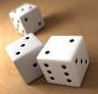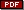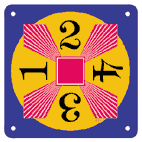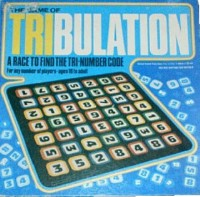More Number Sense Activities## Contig Game

This game challenges and extends the number sense experience students have gained from writing equivalent mathematical expressions for target numbers.   In the Contig game, students toss three dice and use those numbers with any operations to name and capture numbers on the gameboard.   As with all good games, there is a winning strategy that drives students to search for the best option.   In this case, the student gets a point for the captured number and an additional point for every captured number it touches.   These bonus points challenge students to perform many mental combinations and calculations in search of the most points.

DownloadContig Gameboard and Directions## 24 Game

The 24 Game builds on students' ability to find equivalent names for numbers.   Each card presents 4 different digits that students must combine, using any and all operations, depending on the level selected, in a mathematical expression that produces an answer of 24.   This game differs from the Everyday Mathematics Name That Number game in that students must use all four numbers to be successful.

24 Game materials:The original 24 Game was developed by Robert Sun in 1988. Many different versions are now available online or in teacher supply stores.   There are 24 Game tournaments and an online version of the game is currently available on a subscription basis.

Recording Sheet:   A recording sheet has been developed to allow students to record the mathematical expressions they find for each card.   While the 24 Game does not require students to write down the expression, teachers find that this writing is an effective instructional strategy for students learning the game.   Writing down solutions allows students to compare their mathematical expressions and broaden their approach in the process by critiquing the solutions of their classmates.## Tribulation Game

This game also challenges students to find equivalent names for numbers.   In Tribuation, students must search the gameboard for 3 numbers in a row (vertically, horizontally or diagonally as in a word search) that combine to make the target number.   In this game, however, there is a prescribed formula for combining the numbers.   Students must multiply the first two numbers then add or subtract the third number to produce the target number.

Students quickly learn that any string of three numbers produce four different results since the string of numbers can be considered both forwards and backwards as in word searches.   Consider that the numbers 3, 5 and 8 are in a horizontal row.   These three numbers produce the following results when combined in the formula:

• 3 x 5 + 8 = 23
• 3 x 5 - 8 = 7
• 8 x 5 + 3 = 43
• 8 x 5 - 3 = 37

Note that the student may not begin with the middle number.   Once again, students will perform many mental calculations in a search for a viable solution on the gameboard.

This game was originally a board game in the 1980s but has been developed into a classroom game using a gameboard template designed to be inserted in a sheet protector and used with dry-erase pens so that students can easily circle and record solutions.   Two different approaches are effective in using this game:   (1) students search for one solution for 6-8 different target numbers, and (2) students search for many different solutions for one number.   Samples of both types of activities are included in the Tribulation directions packet for teachers.